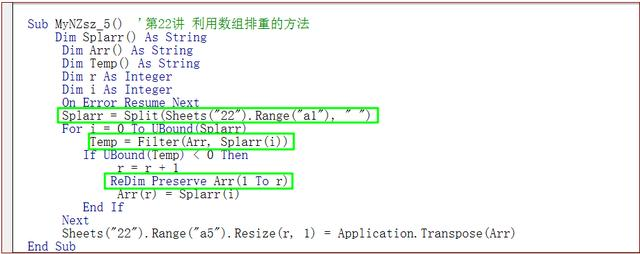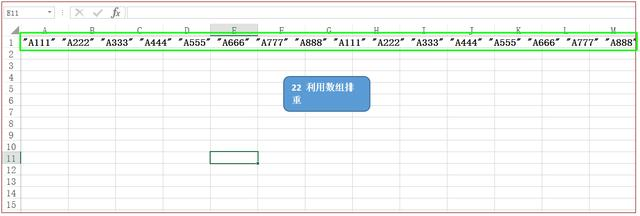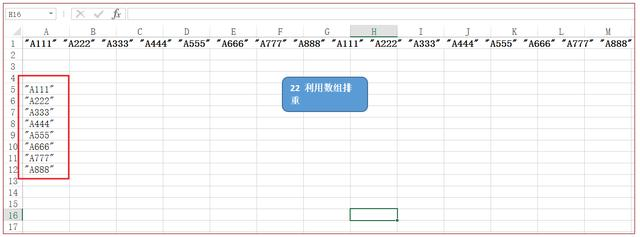• 利用VBA筛选重复数据

千次阅读 2019-06-17 14:30:02
目标：在重复数据中按照一定规则提取、组合。 Sub match() Dim i, j, z, n, flag, A, B, C Set A = Worksheets("Sheet1").UsedRange Set B = Worksheets("Sheet2").UsedRange Set C = Worksheets("Sheet3")....

目标：在重复数据中按照一定规则提取、组合。

Sub match()
Dim i, j, z, n, flag, A, B, C

Set A = Worksheets("Sheet1").UsedRange
Set B = Worksheets("Sheet2").UsedRange
Set C = Worksheets("Sheet3").UsedRange

For i = 3 To A.Rows.Count
For j = 2 To B.Rows.Count
If A.Cells(i, 1) = B.Cells(j, 2) Then
'险种名称
Debug.Print B.Cells(j, 2)
Select Case B.Cells(j, 3)
Case Is = A.Cells(1, 3)
'城镇职工基本医疗保险
'缴费基数  赋值列不同
A.Cells(i, 2) = Val(B.Cells(j, 6))
'缴纳金   赋值列不同
A.Cells(i, 3) = Val(B.Cells(j, 8))
A.Cells(i, 4) = Val(B.Cells(j, 9))
Case Is = A.Cells(1, 6)
'城镇企业职工基本养老保险
'缴费基数
A.Cells(i, 5) = Val(B.Cells(j, 6))
'缴纳金
A.Cells(i, 6) = Val(B.Cells(j, 8))
A.Cells(i, 7) = Val(B.Cells(j, 9))
Case Is = A.Cells(1, 8)
'公务员医疗补助
'缴费基数
A.Cells(i, 5) = Val(B.Cells(j, 6))
'缴纳金
A.Cells(i, 8) = Val(B.Cells(j, 8))
A.Cells(i, 9) = Val(B.Cells(j, 9))
Case Is = A.Cells(1, 10)
'生育保险
'缴费基数
A.Cells(i, 5) = Val(B.Cells(j, 6))
'缴纳金
A.Cells(i, 10) = Val(B.Cells(j, 8))
A.Cells(i, 11) = Val(B.Cells(j, 9))
Case Is = A.Cells(1, 12)
'工伤保险
'缴费基数
A.Cells(i, 5) = Val(B.Cells(j, 6))
'缴纳金
A.Cells(i, 12) = Val(B.Cells(j, 8))
A.Cells(i, 13) = Val(B.Cells(j, 9))
Case Is = A.Cells(1, 14)
'失业保险
'缴费基数
A.Cells(i, 5) = Val(B.Cells(j, 6))
'缴纳金
A.Cells(i, 14) = Val(B.Cells(j, 8))
A.Cells(i, 15) = Val(B.Cells(j, 9))
Case Else

End Select

End If
Next
Next

'把名字找到，然后将属性整合

j = 3
flag = 0
'Debug.Print C.Cells(j, 1)

For i = 3 To A.Rows.Count
If C.Cells(j, 1) = "" Then
For z = 3 To j   'z用于控制待写入数据和前面数据的重复比较次数
'Debug.Print z
If C.Cells(z, 1) = A.Cells(i, 1) Then
If C.Cells(z, 1) <> "" Then
flag = 1   'flag用于判断待写入数据是否和前面的数据重复
Debug.Print z
End If
End If
Next

If flag = 0 Then   '只有经过上面的重复数据循环判断，才能确定这个数据不是重复数据
C.Cells(j, 1) = A.Cells(i, 1)
For n = 2 To 15   'n 用于控制写出数据的列数
C.Cells(j, n) = A.Cells(i, n)
Next
Debug.Print C.Cells(j, 1)
j = j + 1    '
End If

End If
flag = 0
Next

Debug.Print "Done"

End Sub

展开全文• 找出不重复值 Sub 找出选定范围内不重复的值() On Error Resume Next Dim d As Object Set d = CreateObject("scripting.dictionary") For c_i = 1 To selection.Columns.Count For Each ce In selection....

找出不重复值

Sub 找出选定范围内不重复的值()
On Error Resume Next

Dim d As Object
Set d = CreateObject("scripting.dictionary")
For c_i = 1 To selection.Columns.Count
For Each ce In selection.Columns(c_i).Cells
If ce <> "" Then
'D(ce.Value) = ""
If d.Exists(ce.Value) Then
d(ce.Value) = d(ce.Value) + 1
Else
d(ce.Value) = 1
End If
End If
Next
Next
'Debug.Print d.Count
If MsgBox("是否在邻列显示出现次数？", vbYesNo, "统计次数") = vbYes Then
标记 = True
End If
e = InputBox("希望在那个单元格下生成结果：", "结果输出", Chr(97 + selection.Columns(1).Cells(1).Column + 2) & selection.Columns(1).Cells(1).row)
m = Left(e, 1)
jj = Mid(e, 2, 1)
Range(m & jj) = "不重复值"
If 标记 = True Then
Range(Chr((Asc(m) + 1)) & jj) = "频率" '大于1的值
End If
For Each Key In d.Keys
Range(m & (jj + 1)) = Key
If 标记 = True Then
'            If d(Key) > 1 Then Range(Chr((Asc(m) + 1)) & (jj + 1)) = d(Key)
Range(Chr((Asc(m) + 1)) & (jj + 1)) = d(Key)
End If
jj = jj + 1
Next
Set d = Nothing
'最后进行排序
'     Range(m & selection.Columns(1).Cells(1).row & ":" & Chr((Asc(m) + 1)) & (jj)).Select
Range(e & ":" & Chr((Asc(m) + 1)) & (jj)).Sort Key1:=Range(m & Mid(e, 2, 1) + 1), Order1:=xlAscending, HEADER:=xlYes _
, OrderCustom:=1, MatchCase:=False, Orientation:=xlTopToBottom, _
SortMethod:=xlPinYin, DataOption1:=xlSortNormal
End Sub

展开全文VBA excel vba
• EXCEL如何实现动态筛选不重复记录复制到新列中 求一函数公式：工作表中有几千条记录，希望从中查找中不重复的记录复制到新列中，即无论某一记录有一条或多条（当在“现状”列中加两笔相同的F，“结果”列中则只Show...excel 函数公式
• excel筛选重复姓名 求出Excel中不重复的人的姓名的VBA代码，看到标题你是不是很兴奋，这不就是你想学习的知识吗？当你掌握excel筛选重复姓名这个知识的时候你一定很兴奋，一定不会后悔看了excel筛选重复姓名 求出...excel筛选重复姓名 求出Excel中不重复的人的姓名的VBA代码，看到标题你是不是很兴奋，这不就是你想学习的知识吗？当你掌握excel筛选重复姓名这个知识的时候你一定很兴奋，一定不会后悔看了excel筛选重复姓名 求出Excel中不重复的人的姓名的VBA代码这篇文章的，因为你确实把这个问题解决了，不是吗？

前面的文章我们学习了关于统计重复的姓名的问题，现在，我们反其道而行之，学习一下求出Excel中不重复的人的姓名的VBA代码。

当然，还是使用VBA中的字典来处理。假设有3张工作表，每张表格的A列都是姓名列，所有这些姓名中有些是重复的，要求编写一段代码，在另一个工作表上显示不重复的姓名。

使用VBA的话，代码如下：

Dim i&, Myr&, Arr

Dim d, k, t, Sht As Worksheet

Set d = CreateObject("Scripting.Dictionary")

For Each Sht In Sheets

If Sht.Name <> "Sheet4" Then

Myr = Sht.[a65536].End(xlUp).Row

Arr = Sht.Range("a2:a" & Myr)

For i = 1 To UBound(Arr)

d(Arr(i, 1)) = ""

Next

End If

Next

k = d.keys

Sheet4.[a3].Resize(d.Count, 1) = Application.Transpose(k)

Set d = Nothing

部分代码解释：

1、For Each Sht In Sheets ：For Each…Next循环结构，这种形式是VBA特有的，用于对对象的循环非常适用。意思是在所有的工作表中依次循环。

2、If Sht.Name <> "Sheet4" Then ：如果这个工作表的名字不等于”Sheet4”时执行下面的代码。

3、Myr = Sht.[a65536].End(xlUp).Row ：求得这个工作表A列有数据的最后一行的行数，把它赋给变量Myr。这里用了长整型数据类型(Long)，数据范围最大可到2,147,483,647，是为了避免数据很多的时候会超出整型数据类型(Integer)而出错，因为整型数据类型数据范围最大只到32,767。

4、Arr = Sht.Range("a2:a" & Myr) ：把A列数据赋给数组Arr。

5、For i = 1 To UBound(Arr) ：For…Next循环结构，从1开始到数组的最大上限值之间循环。Ubound是VBA函数，返回数组的指定维数的最大值。

6、d(Arr(i, 1)) = “” ：这句代码的意思就是把关键字Arr(i,1)加入字典，关键字对应的项为空，相当于字典中的这个关键字没有解释。和d.Add Arr(i,1), ""的效果相同，只是代码更简洁一些。

7、k=d.keys ：把字典d中存在的所有的关键字赋给变量k。得到的是一个一维数组，下限为0，上限为d.Count-1。Keys是字典的方法，前面已经讲过了。

8、Sheet4.[a3] .Resize(d.Count, 1) = Application.Transpose(k) ：把字典d中所有的关键字赋给表4以a3单元格开始的单元格区域中。

以上就是excel筛选重复姓名 求出Excel中不重复的人的姓名的VBA代码全部内容了，希望大家看完有所启发，对自己的工作生活有所帮助，想要了解更多跟excel筛选重复姓名 求出Excel中不重复的人的姓名的VBA代码请关注我们优词网！展开全文• 大家好，我们今日继续讲解VBA数组与字典解决方案第22讲：在VBA中如何使用动态数组，以及利用动态数组去除重复值的方法。如果文本中含有大量的重复值，此时，如果我们要剔除重复值，该怎么办？用VBA的方法该如何做到...

大家好，我们今日继续讲解VBA数组与字典解决方案第22讲：在VBA中如何使用动态数组，以及利用动态数组去除重复值的方法。如果文本中含有大量的重复值，此时，如果我们要剔除重复值，该怎么办？用VBA的方法该如何做到呢？我在这讲和下一讲中将解答这个问题，并提供给读者一个可以测试的实例。今日先讲这个内容要用到的知识点。

一 ：Filter函数：这个函数返回一个下标从零开始的数组，该数组包含基于指定筛选条件的一个字符串数组的子集，语法如下：Filter(sourcesrray, match[, include[, compare]])

参数

a) sourcesrray是必须的，要执行搜索的一维字符串数组。

b) match是必须的，要搜索的字符串。

c) include是可选的，Boolean值，表示返回子串是否包含match字符串。如果参数include是True，Filter函数返回的是包含match参数子字符串的数组子集。如果参数include是False，Filter函数返回的是不包含match参数子字符串的数组子集。

d) compare是可选的，所使用的字符串比较类型。

二：ReDim语句，这个语句在过程级别中使用，用于为动态数组变量重新分配存储空间，语法如下：

ReDim [Preserve] varname(subscripts) [As type] [, varname(subscripts) [As type]]

参数

a) Preserve是可选的，关键字，当改变原有数组最末维的大小时，使用此关键字可以保持数组中原来的数据。

b) varname是必须的，变量的名称。

c) subscripts是必须的，数组变量的维数，最多可以定义 60 维的多维数组，使用下面的语法；[lower To] upper [,[lower To] upper]

使用动态数组去除文本中的重复值，如下面的代码所示。

Sub MyNZsz_5() '第22讲 利用数组排重的方法

Dim Splarr() As String

Dim Arr() As String

Dim Temp() As String

Dim r As Integer

Dim i As Integer

On Error Resume Next

Splarr = Split(Sheets("22").Range("a1"), " ")

For i = 0 To UBound(Splarr)

Temp = Filter(Arr, Splarr(i))

If UBound(Temp) < 0 Then

r = r + 1

ReDim Preserve Arr(1 To r)

Arr(r) = Splarr(i)

End If

Next

Sheets("22").Range("a5").Resize(r, 1) = Application.Transpose(Arr)

End Sub

代码解析：

MySplitarr过程将工作表Sheets("22")中A1单元格的文本去除重复值后写入到工作表Sheets("22")中的A列单元格。

第2行代码声明数组Splarr用来保存Sheets("22")中A1单元格的文本。

第3行代码声明数组Arr用来保存去除重复值后的文本。

第4行代码声明数组Temp用来判断文本是否重复。

第5行代码声明变量r用来保存去除重复值后的文本数量。

第7行代码启动错误处理程序来忽略错误，因为在程序运行到第11行代码会发生下标越界错误。

第8行代码使用Split 函数以Sheets("22")中A1单元格的文本创建一个下标从零开始的一维数组。

第9行代码使用For...Next语句遍历数组Splarr的所有元素。

第10行代码使用Filter函数创建一个数组Temp用来保存以当前Splarr数组的值在Arr数组中的搜索结果。

第11行代码根据返回的数组Temp的最大下标来判断当前Splarr数组的值是否重复。在使用使用Filter函数时如果没有相匹配的值，将返回一个空数组，最大下标小于0。如果没有找到，返回的结果是-1.

第12行代码如果当前Splarr数组的值不重复则将变量r的值加1。

第13行代码重新定义动态数组大小。

第14行代码将不重复值添加到数组Arr中。

第15行代码使用工作表Transpose函数将去除重复值的的文本转置后写入到工作表单元格中。

代码的截图：运行前的截图：很显然有很多的重复数值：运行后的截图：今日内容回向：

1 上述代码是否清楚了它的运行过程呢？

2 第7行代码启动错误处理程序来忽略错误，这是为什么呢？

展开全文• excel vba筛选

万次阅读 2017-02-10 09:45:32
1、筛选vba 代码为 set rg=range("a1:a100") rg.AutoFilter Field:=1, Criteria1:="内容" rg 为筛选区域， Criteria1为筛选的值，比如在A1列内筛选内容为北京区的 ，则 Criteria1="北京区" 2、高级筛选 ...excel筛选 vba筛选
• VBA 是一种很久远的编程语言，但并过时。在满足以下两个条件时，借助 VBA 可以极大的提升生产率，降低出错率：你的电脑上允许自主安装软件； 你需要执行的工作中大部分的步骤都是固定且重复的。项目背景近期接到...vba移动文件
• 问题大概是这样的，一个很大的Excel文件，其中有些行是重复的，也就是说，有2行是完全一样的，而有些行是不重复的，现在的问题是要找出所有不重复或者重复的行，你没有听明白。你大概考虑了一下，用“VLOOKUP”查找...
• 不同表格查找重复数据VBA

千次阅读 2018-12-25 13:50:54
分析两个表格中对应列的重复情况。 Sub Match_Dec() '两个表格，表格中的某一列为对应列，查找这两列中的重复记录和差异记录。 Dim ar As Long, br As Long, i As Integer, j As Integer, num As Integer 'ar/...
• 我有一个很大的代码,它接受用户选择的参考号,并通过过滤和复制数据在多个其他工作表上定位相应的行(可以有多个行,也可以没有行)。这很好,除了它复制所有可见数据(列A-N)当我真的希望它复制列A到K时(因为粘贴表上的L...
• 大家好，我们今日继续讲解VBA代码解决方案的第61讲内容：在VBA中如何使用动态数组，以及利用动态数组去除重复值的方法。在上一讲中我们讲了使用数组函数将单元格中的文本进行分隔后写入到工作表中的方法，那么问题...
• 第一种情况保留不重复的记录行，重复的只保留一行。 1、打开有重复数据的EXCEL 2、Alt+F11 打开宏的VB编辑器 3、左边双击：ThisWorkBook 4、贴入以下代码并运行即可： Sub 删除重复数据() '删除col列的重复...excel vba integer string header
• Range.AutoFIlter方法可以进行自动筛选，语法结构如下： Range.AutoFilter(Field,Criteria1Operator,Criteria2,VIsibleDropDown); 参数说明： Field可选，筛按33选的字段的整型偏移量，；例如筛选A列的字段则取值...Excel VBA 自动筛选
• excel sql语句筛选重复数据 使用SQL语句进行数据筛选的方法，这个问题是我们很多人都很困惑的一个问题，excel sql语句筛选重复数据是我们非常常用的一个东西，也是我们工作和学习中必可少的一个数据公式，那么具体...
• 之前做过用用VBA筛选数据，去重的相关工作，但是后面给忘记了，最近需要用到，又重新写了一个。鉴于此，还是做一个归纳，以后再需要的时候也方便一些。 VBA主要用在Excel中，别的就说了，只说Excel中的情况 一个...编程语言 vba excel
• 如果局限于一种方法，去探索其他方法，往往会错失一些机会。想想看，如果你能用一半的时间完成你手头的工作，节省下来的时间可以做很多有意义的事情的。通过自动化，让人们从一些简单重复的劳动中解放出来，投入到...
• VBA数据透视表的创建

千次阅读 2021-01-21 15:21:37
方法 读/写 值类别 默认值 说明 .ColumnGrand ...数据透视表在刷新或移动域时自动设置格式 ...如果数据透视表在有错误的单元格中显示用户自定义的错误字符串，则该值为 True。 .ErrorString 返回...excel vba
• 1.去除重复项 2.自动生成下拉菜单 3.重复项自动求和 附上代码： Private Sub Worksheet_SelectionChange(ByVal Target As Range) Dim d1 As Object, d2 As Object, d3 As Object, arr, i As Integer, k, brr, ...excel vba 算法 实时搜索
• 如何利用VBA数组提取该列不重复的值，并存放于新表中？ 日期 销售经理 客户分类 区域 分配业务员 回款地 所属公司 产品分类 客户名称 销售数量 单价 销售金额 送货单号 2010...
• 如图所示，本例希望能够从员工列表中随机抽取5名员工的记录，该如何用VBA实现？ 员工编号 姓名 部门 员工编号 姓名 部门 0006 程建华 管理部 0314 张宏 销售部 0009 ...
• 我在ACEESS做了一个窗体,内含一个子窗体，子窗体的控件来源是一个没有筛选条件的查询，在窗体里用VBA生成子窗体筛选条件。例：在窗体输入筛选条件后，子窗体筛选结果为：客户 字段1 字段2 ....(子窗体页眉)A 1 2B 0 ...
• 1 combobox控件，显示A列处理后的数据，求助问题内容 QQ群玩家求助的内容， 说是这样报错 看了下，代码有问题 b=0 一般应该在循环内，并且redim preserve 数组估计被不断重置了 另外combobox1.list 报错...
• Public Sub Filter() ' sheet1 是从乾坤里导出的总表 ...' 只要把已经开票的提单号拷贝到sheet2 的E列就可以了，sheet1 从乾坤导出来的格式变化，唯一要做的就是在sheet1里的S列添加一个标题filter
• excel vba数据分析

千次阅读 2019-01-20 12:20:00
VBA(Visual Basic for Application)是Microsoft Office系列软件的内置编程语言，其语法结构与Visual Basic编程语言互相兼容，采用的是面向对象的编程机制和可视化的编程环境。 第一节 标识符 一．定义 标识符是一...
• 使用VBA添加ListObject对象 Excel中数据存储的结构 (1)用输入界面的表格或者汇总形式的表格直接代替数据库存储。 (2)使用多维表（交叉表）记录数据。 示例 如图所示，该表为某公司供应商信息录入表以及供应商...
• 展开全部公式：b10输入=CONCATENATE(LARGE(A1:A10,1),",",LARGE(A1:A10,2),",",LARGE(A1:A10,3),",",LARGE(A1:A10,4))，然后向下填充即可vba：Sub宏1()Fori=10ToRange("a65536").End(xlUp).Rowss=""Forj=1To4Ifss&l.......